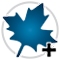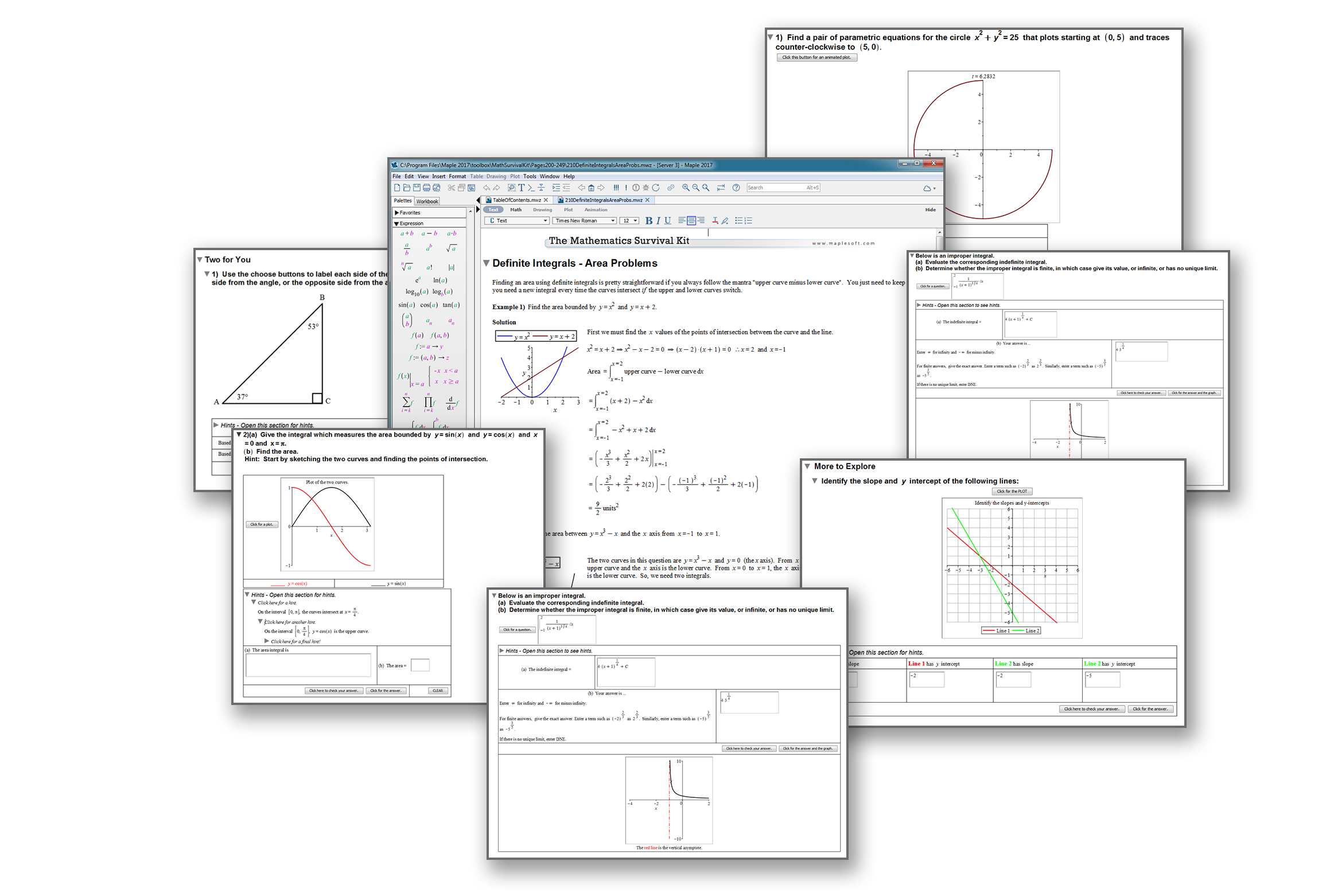The Mathematics Survival Kit - New Edition- MaplesoftThe Mathematics Survival Kit

New Edition!

The latest release of The Mathematics Survival Kit - Maple Edition contains 31 additional topics, bringing the total to 171. All of the new topics were created in response to requests from readers of earlier editions. The additional content deepens the coverage of mathematical topics at every level, from early high school to university, and even includes some new pre-high school topics. The additional content follows the same structure as the rest of the book, with explanations, examples, and automatic grading of randomly generated practice questions for every topic.

New material includes:

• Operations with decimals
• Ratio, rate, and percent
• Complex numbers
• Partial fractions
• Trigonometric ratios: SOH CAH TOA
• Graphing straight lines and solving linear equations
• Composite functions
• L’Hôpital’s Rule
• Integration by trig substitution
• Definite integral problems
• Integrating functions with discontinuities
• Differential equations - separation of variables
• Parametric equations
• Probability
• Proof by induction
• and more!